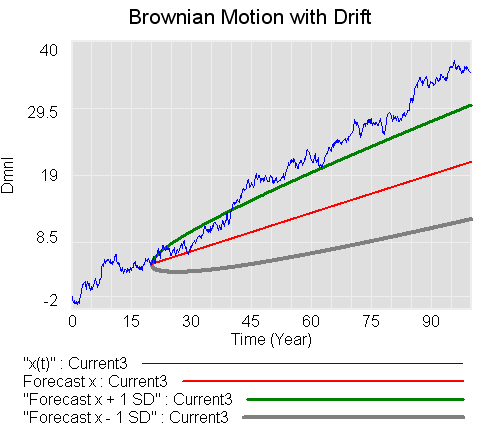## Randomness in System Dynamics

A discrete event simulation guru asked Ventana colleague David Peterson about the representation of randomness in System Dynamics models. In the discrete event world, granular randomness is the whole game, and it often doesn’t make sense to look at something without doing a lot of Monte Carlo experiments, because any single run could be misleading. The reply:

• Randomness 1:  System Dynamics models often incorporate random components, in two ways:
• Internal:  the system itself is stochastic (e.g. parts failures, random variations in sales, Poisson arrivals, etc.
• External:  All the usual Monte-Carlo explorations of uncertainty from either internal randomness or via replacing constant-but-unknown parameters with probability distributions as a form of sensitivity analysis.
• Randomness 2:  There is also a kind of probabilistic flavor to the deterministic simulations in System Dynamics.  If one has a stochastic linear differential equation with deterministic coefficients and Gaussian exogenous inputs, it is easy to prove that all the state variables have time-varying Gaussian densities.  Further, the time-trajectories of the means of those Gaussian process can be computed immediately by the deterministic linear differential equation which is just the original stochastic equations, with all random inputs replaced by their mean trajectories.  In System Dynamics, this concept, rigorous in the linear case, is extended informally to the nonlinear case as an approximation.  That is, the deterministic solution of a System Dynamics model is often taken as an approximation of what would be concluded about the mean of a Monte-Carlo exploration.  Of course it is only an approximate notion, and it gives no information at all about the variances of the stochastic variables.
• Randomness 3:  A third kind of randomness in System Dynamics models is also a bit informal:  delays, which might be naturally modeled as stochastic, are modeled as deterministic but distributed.  For example, if procurement orders are received on average 6 months later, with randomness of an unspecified nature, a typical System Dynamics model would represent the procurement delay as a deterministic subsystem, usually a first- or third-order exponential delay.  That is the output of the delay, in response to a pulse input, is a first- or third-order Erlang shape.  These exponential delays often do a good job of matching data taken from high-volume stochastic processes.
• Randomness 4:  The Vensim software includes extended Kalman filtering to jointly process a model and data, to estimate the most likely time trajectories of the mean and variance/covariance of the state variables of the model. Vensim also includes the Schweppe algorithm for using such extended filters to compute maximum-likelihood estimates of parameters and their variances and covariances.  The system itself might be completely deterministic, but the state and/or parameters are uncertain trajectories or constants, with the uncertainty coming from a stochastic system, or unspecified model approximations, or measurement errors, or all three.

“Vanilla” SD starts with #2 and #3. That seems weird to people used to the pervasive randomness of discrete event simulation, but has a huge advantage of making it easy to understand what’s going on in the model, because there is no obscuring noise. As soon as things are nonlinear or non-Gaussian enough, or variance matters, you’re into the explicit representation of stochastic processes. But even then, I find it easier to build and debug  a model deterministically, and then turn on randomness. We explicitly reserve time for this in most projects, but interestingly, in top-down strategic environments, it’s the demand that lags. Clients are used to point predictions and take a while to get into the Monte Carlo mindset (forget about stochastic processes within simulations). The financial crisis seems to have increased interest in exploring uncertainty though.

## Stochastic Processes

This model replicates a number of the stochastic processes from Dixit & Pindyck’s Investment Under Uncertainty. It includes Brownian motion (Wiener process), geometric Brownian motion, mean-reverting and jump processes, plus forecast confidence bounds for some variations.Units balance, but after updating this model I’ve decided that there may be a conceptual issue, related to the interpretation of units in parameters of the Brownian process variants. This arises due to the fact that the parameter sigma represents the standard deviation at unit time, and that some of the derivations gloss over units associated with substitutions of dz=epsilon*SQRT(dt). I don’t think these are of practical importance, but will revisit the question in the future. This is what happens when you let economists get hold of engineers’ math. 🙂

These structures would be handy if made into :MACRO:s for reuse.

stochastic processes 3.mdl (requires an advanced version of Vensim)

stochastic processes 3.vpm (published package; includes a sensitivity setup for varying NOISE SEED)

stochastic processes 3 PLE.mdl (Runs in PLE, omits only one equation of low importance)